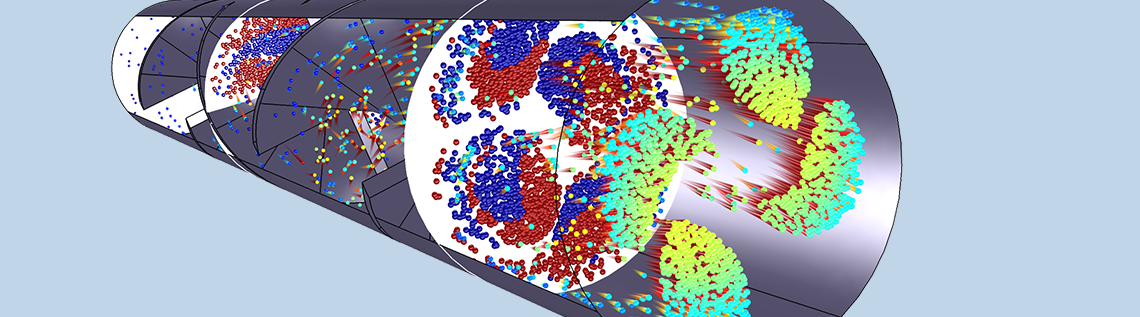# 模拟流体中的粒子时应该使用哪种公式？

2020年 12月 4日

### 示例：小球形粒子的重力沉降

(1)

\frac{\textrm{d}}{\textrm
{d}t}\left(m_\textrm{p}\frac{\textrm{d}
\mathbf{q}}{\textrm{d}t}\right) = \mathbf{F}_\textrm{t}

• mp（SI单位：kg）是粒子的质量
• q（SI单位：m）是粒子的位置矢量
• Ft（SI单位：N）是作用在粒子上的净力或总力

(2)

\mathbf{F}_\textrm{g} = \frac{\rho_\textrm{p}-\rho}{\rho_\textrm{p}}m_\textrm{p}\mathbf{g}
\mathbf{F}_\textrm{D} = 3\pi \mu d_\textrm{p}\left(\mathbf{u}-\mathbf{v}\right)

• ρp（SI单位：kg/m3）是粒子的密度
• ρ（SI单位：kg/m3）是周围流体的密度
• g（SI单位：m/s2）是重力引起的加速度（海平面以下约为 9.8m/s2
• μ（SI单位：Pa s）是周围流体的动力黏度
• dp（SI单位：m）是粒径
• u（SI单位：m/s）是周围流体的速度
• v（SI单位：m/s）为粒子的速度（v≡dq/dt

\textrm{Re}_\textrm{r} \equiv \frac{\rho d_\textrm{p}\left|\mathbf{u}-\mathbf{v}\right|}{\mu} \ll 1

(3)

m_\textrm{p} = \frac{\pi}{6}\rho_\textrm{p}d_\textrm{p}^3

(4)

\frac{\textrm{d}^2 \mathbf{q}}{\textrm{d}t^2}
=
\frac{\rho_\textrm{p}-\rho}{\rho_\textrm{p}}\mathbf{g}
+
\frac{1}{\tau_\textrm{p}}\left(\mathbf{u}-\mathbf{v}\right)

\tau_\textrm{p} \equiv \frac{\rho_\textrm{p}d_\textrm{p}^2}{18\mu}

τp具有时间单位，并且通常被称为拉格朗日时间尺度（Lagrangian time scale粒子速度响应时间（particle velocity response time，下面我们将解释其原因。

(5)

\frac{\textrm{d}^2 q_y}{\textrm{d}t^2}
=
-\frac{\rho_\textrm{p}-\rho}{\rho_\textrm{p}}g-\frac{1}{\tau_\textrm{p}}v_y

\begin{aligned}\\
q_y &= -v_\textrm{t}\left\{t+\tau_\textrm{p}\left[\exp\left(-\frac{t}{\tau_\textrm{p}}\right)-1\right]\right\}\\
v_y &= -v_\textrm{t}\left[1-\exp\left(-\frac{t}{\tau_\textrm{p}}\right)\right]\\
\end{aligned}

v_\textrm{t} \equiv \tau_\textrm{p}g\frac{\rho_\textrm{p}-\rho}{\rho_\textrm{p}}

### 转换为无量纲变量

\begin{aligned}q_y^{\prime} &= t^{\prime} – \exp\left(-t^{\prime}\right)+1\\
v_y^{\prime} &= -1 + \exp\left(-t^{\prime}\right)\\\end{aligned}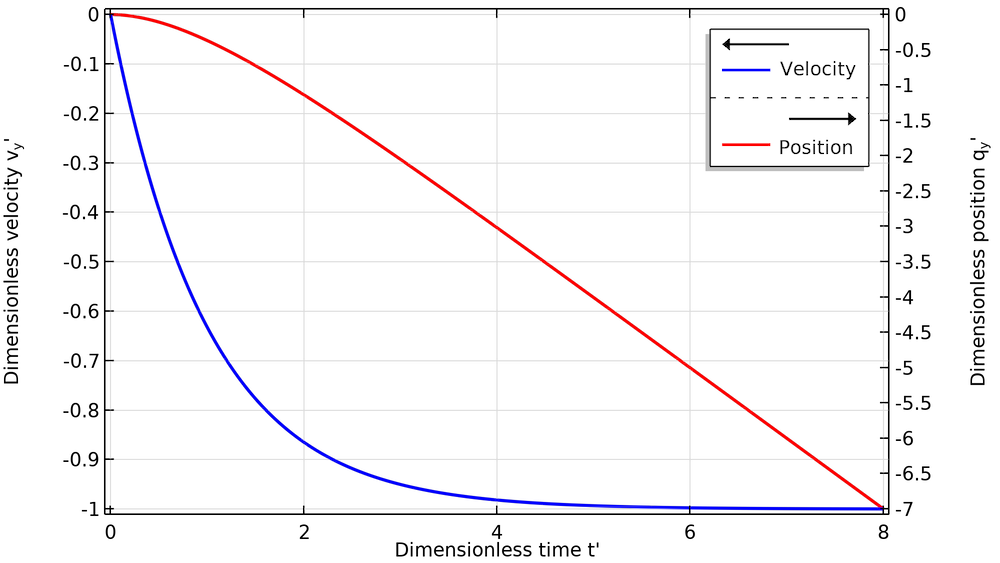### 一些典型粒径的时间尺度

1 1.009×10-3 998.2 1.2×10-7 6.5×10-7
20 1.009×10-3 998.2 4.8×10-5 2.6×10-4
50 1.009×10-3 998.2 3.0×10-4 1.6×10-3

τp 和直径平方呈线性关系意味着大粒子比小粒子具有更长的速度响应时间和更大的自由沉降速度。这会产生两个主要结果：

1. 大粒子掉落到地面的速度比小粒子快得多。
2. 当大粒子以一定的初始速度射入流体时，会沿着入射轨迹，能够在曳力使它们减速之前行进相当长的距离。相反，较小的粒子将更快地匹配流体速度。当它们散开时，很可能是由于周围流体的湍流扩散所致。

### 数值粒子跟踪仿真

COMSOL Multiphysics® 中的粒子追踪模块提供了一个流体流动颗粒跟踪 接口，该接口通过数值求解牛顿第二定律来模拟周围流体中单个粒子的运动。基本上，此接口可求解方程1，同时允许我们向方程右侧添加各种不同的力。它还包括用于设置初始粒子位置和速度以及检测和处理粒子与周围几何中的表面的碰撞的各种选项。

### 处理小粒子和长时间尺度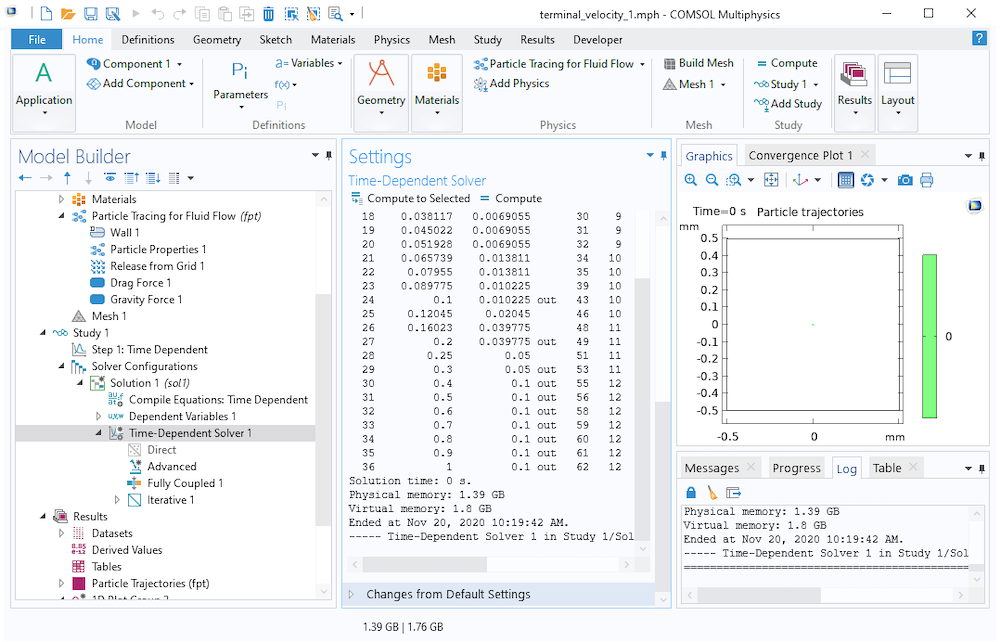### 粒子追踪模型中的数值刚度处理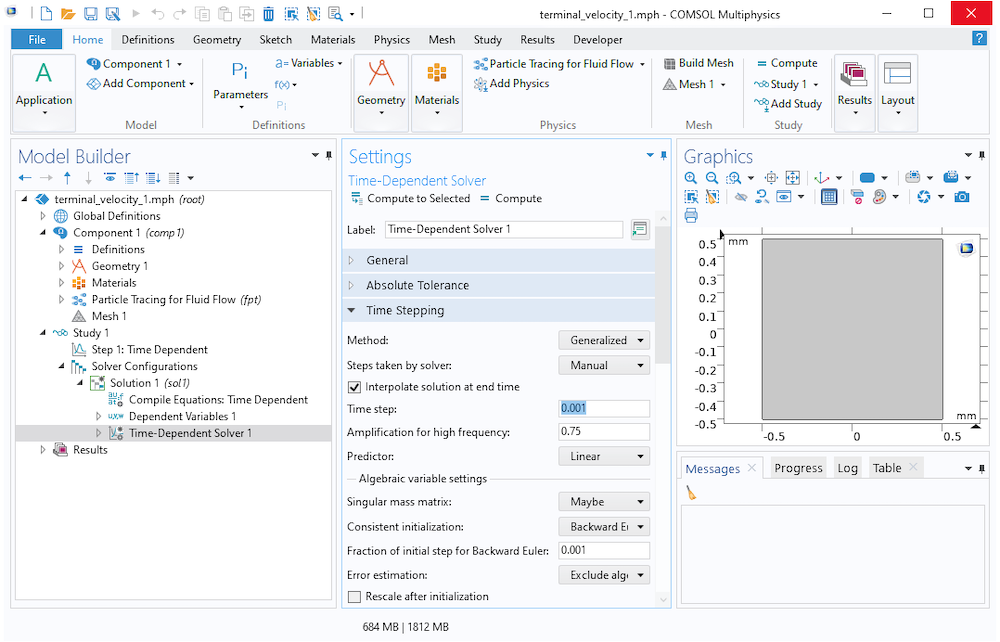\begin{aligned}
\frac{\textrm{d} \mathbf{q}}{\textrm{d}t} &= \mathbf{v}\\
\frac{\textrm{d} \mathbf{v}}{\textrm{d}t} &= \frac{\rho_\textrm{p}-\rho}{\rho_\textrm{p}}\mathbf{g} + \frac{1}{\tau_\textrm{p}}\left(\mathbf{u}-\mathbf{v}\right)\\
\end{aligned}

(6)

\frac{\rho_\textrm{p}-\rho}{\rho_\textrm{p}}\mathbf{g}
+
\frac{1}{\tau_\textrm{p}}\left(\mathbf{u}-\mathbf{v}\right)
=
\mathbf{0}

\mathbf{v} = \tau_\textrm{p}\frac{\rho_\textrm{p}-\rho}{\rho_\textrm{p}}\mathbf{g}+\mathbf{u}

(7)

\mathbf{v} = \frac{\tau_\textrm{p}}{m_\textrm{p}}\mathbf{F}_\textrm{other}+\mathbf{u}

• 牛顿型：求解方程1
• 牛顿型，一阶：将方程1 分离为 qv 的一对耦合一阶方程，然后求解它们
• 牛顿型，忽略惯性项（自版本 5.6 起可用）：使用方程7 定义速度的简化公式，然后求解 q
• 无质量：一种更简化的公式，其中直接指定 v 来求解 q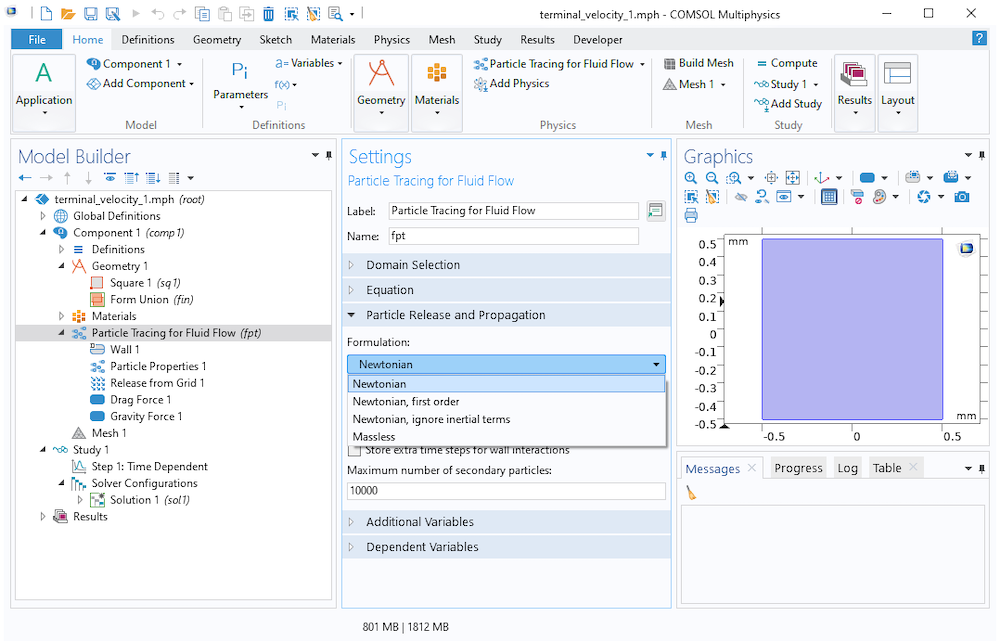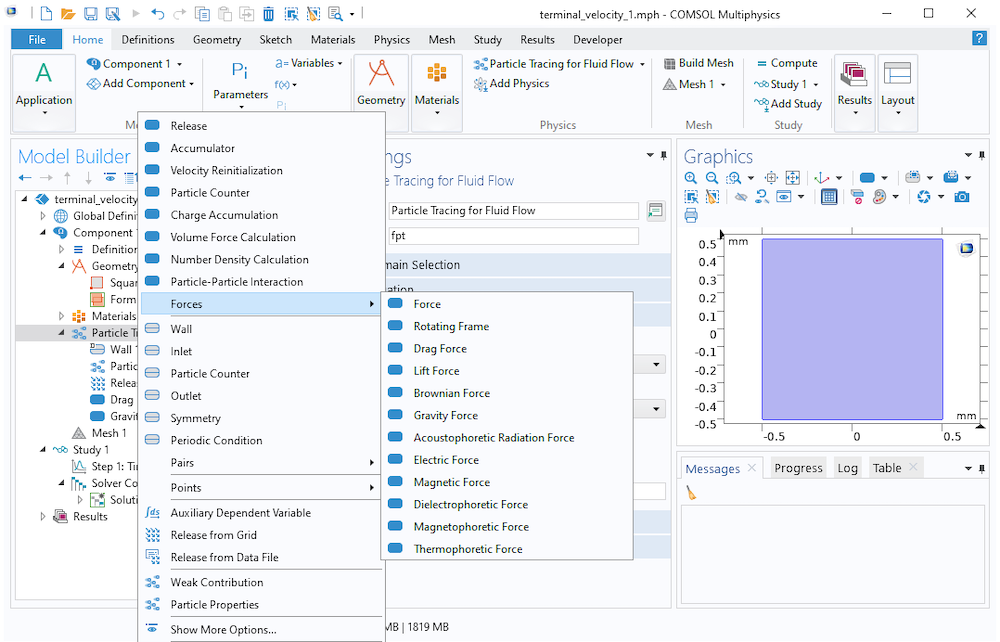### 结语

\tau_\textrm{p} \equiv \frac{\rho_\textrm{p}d_\textrm{p}^2}{18\mu}